Study Guide

# Ratios & Percentages - Converting Decimals to Fractions

## Converting Decimals to FractionsUp top we see forty-five hundredths, which means 45 out of 100. If we reduce that fraction, we get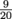.

When converting a decimal to a fraction, all we really need to do is look at the last place value of the decimal and put the right amount of zeros in the denominator. Oh, and then reduce the fraction if possible. To convert 0.9 to a fraction, we look at the last digit's place value, which is that 9 in the tenths place. Then we put the digit 9 over 10 (for tenths place) to get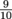.

To convert 0.03 to a fraction, we look at the last digit, which is in the hundredths place. Now we put the digit 3 over 100 to get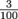.

One more. To convert 5.376 into a fraction, we look at the last place value of the decimal, which is in the thousandths place. Now put all of the digits over 1000 to get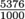. This reduces to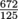.